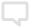隐私设置
10 zjfelinor521TA的下载次数 24

### OFDM—MIMO系统的matlab程序

MIMO OFDM Simulator: OFDM.m: OFDM Simulator (outer function) create_channel.m: Generates a Rayleigh fading frequency-selective channel, parametrized by the antenna configuration, the OFDM configuration, and the power-delay profile. svd_decompose_channel.m: Since full channel knowledge is assumed, transmission is across parallel singular value modes. This function decomposes the channel into these modes. BitLoad.m: Apply the bit-loading algorithm to achieve the desired bit and energy allocation for the current channel instance. ComputeSNR.m: Given the subcarrier gains, this simple function generates the SNR values of each channel (each singular value on each tone is a separate channel). chow_algo.m: Apply Chow's algorithm to generate a particular bit and energy allocation. EnergyTableInit.m: Given the SNR values, form a table of energy increments for each channel. campello_algo.m: Apply Campello's algorithm to converge to the optimal bit and energy allocation for the given channel conditions. ResolvetheLastBit.m: An optimal bit-loading of the last bit requires a unique optimization. modulate.m: Modulate the random input sequence according to the bit allocations for each channel. ENC2.mat: BPSK Modulator ENC4.mat: 4-QAM Modulator (Gray coded) ENC16.mat: 16-QAM Modulator (Gray coded) ENC64.mat: 64-QAM Modulator (Gray coded) ENC256.mat: 256-QAM Modulator (Gray coded) precode.m: Precode the transmitted vector at each time instance by filtering the modulated vector with the right-inverse of the channel's right singluar matrix. ifft_cp_tx_blk.m: IFFT block of the OFDM system. channel.m: Apply the channel to the OFDM frame. fft_cp_rx_blk.m: FFT block of the OFDM system. shape.m: Complete the diagonalization of the channel by filtering the received vector with the left-inverse of the channel's left singular matrix. demodulate.m: Perform a nearest neighbor search knowing the transmit constellation used.

2011-11-05

暂无奖章